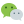# 稀疏数组

## 概念

``````var arr = new Array(3);
console.log(arr);
// (3) [empty × 3]
``````

``````var arr = [1,,2];
console.log(arr);
// (3) [1, empty, 2]
``````

## 特点

``````var arr1 = [1, 2, 3];  // 正常数组
var arr2 = new Array(3);  // 稀疏数组
var arr3 = [1, , 3];  // 稀疏数组

console.log(arr1.length, arr2.length, arr3.length);
// 3 3 3
console.log(arr2, arr3);
// undefined undefined
for (var i = 0; i < 3; i++) {
console.log(arr1[i], arr2[i], arr3[i]);
}
// 1 undefined 1
// 2 undefined undefined
// 3 undefined 3
arr1.forEach(function(x){
console.log(x);
});
// 1
// 2
// 3
arr2.forEach(function(x){
console.log(x);
});
// （无输出）
arr3.forEach(function(x){
console.log(x);
});
// 1
// 3
console.log(0 in arr3, 1 in arr3);
// true false
``````

``````console.time('one');
// 密集数组
Array(...Array(1e5)).forEach(function(){
;
});
console.timeEnd('one');

console.time('two');
// 稀疏数组
Array(1e5).forEach(function(){
;
});
console.timeEnd('two');

// one: 26.3759765625ms
// two: 5.701171875ms
``````

# 密集数组

## 概念

``````var arr1 = [1, 2, 3];
var arr2 = new Array(1, 2, 3);
arr2.forEach(function(x){
console.log(x);
});
// 1
// 2
// 3
``````

## 区别

``````var arr1 = [undefined, undefined, undefined];
var arr2 = new Array(3);
console.log(arr1, arr2);
// undefined undefined
arr1.forEach(function(x){
console.log(x);
})
// undefined
// undefined
// undefined
arr2.forEach(function(x){
console.log(x);
})
// （无输出）
``````

## 拓展

``````var arr1 = new Array(3).fill(1);
console.log(arr1);
// [1, 1, 1]

var arr2 = Array.fill().map((x, i) => i);
console.log(arr2);
// [0, 1, 2]

var arr3 = Array.apply(null, Array(3));
console.log(arr3);
// [undefined, undefined, undefined]
// 这样声明的是密集数组，不是稀疏的

var arr4 = new Array(4).join('a').split('');
console.log(arr4);
// ['a', 'a', 'a']
// 注意定义数组长度比输出数组大 1
``````

### 评论：微信公众号：程序骑士